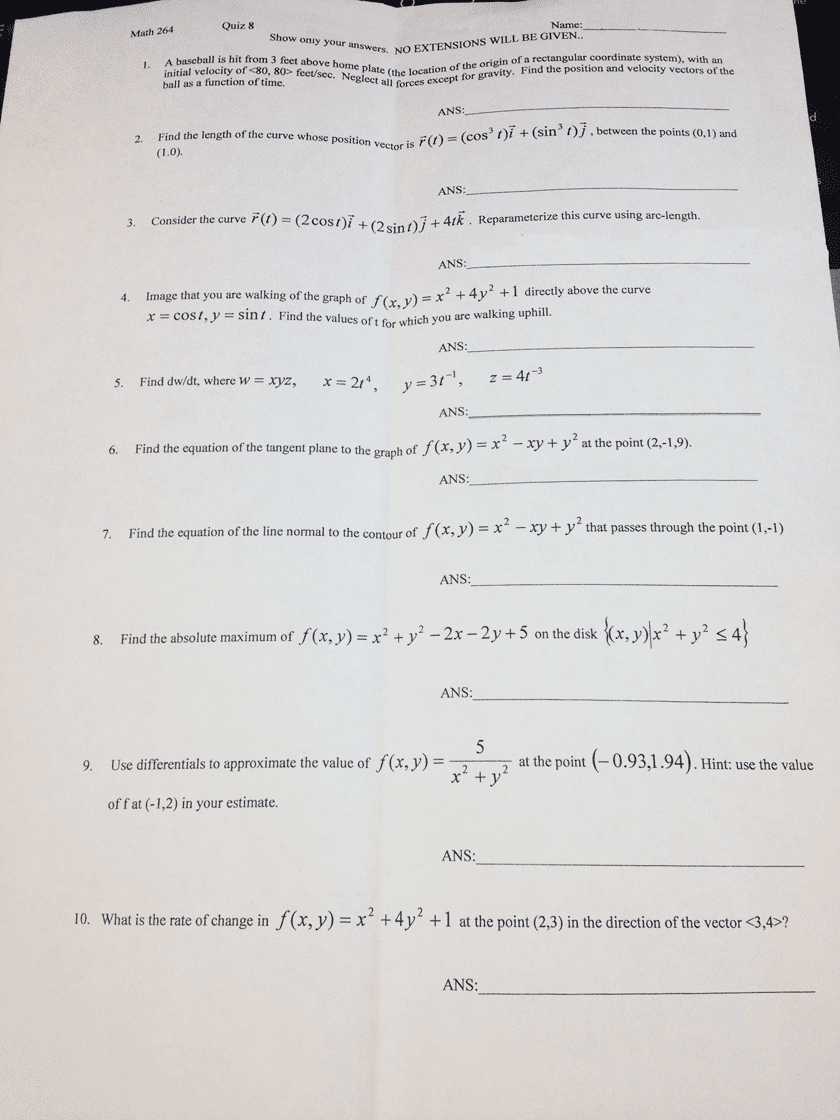1
0
watching
148
views10 Nov 2019

# Calculus 3 help. This is a take home, open book, quiz, but Ineed help on it! I am mainly stuck on the last 4. If I could justget steps on how to approach the answers, that would help me a lot!Thank you in advance!A baseball is hit from 3 feet above home plate (the location of the origin of a rectangular coordinate system), with an initial velocity of feet/sec. Neglect all forces except for gravity. Find the position and velocity vectors of the ball as a function of time. Find the length of the curve whose position vector is r rightarrow (t) = (cos3 t)j rightarrow + (sin3 t)j rightarrow, between the points (0, 1) and (1.0). Consider the curve r rightarrow (t) = (2 cost)I rightarrow + (2sin t)j rightarrow + 4tk rightarrow. Reparameterize this curve using arc-length. Image that you are walking of the group of f (x, y) = x2 + 4y2 + 1 directly above the curve x = cost, y = sin t. Find the values of t for which you are walking uphill. Find dw/dt, where w = xyz, x = 2t4, y = 3t-1, z = 4t-3 Find the equation of the tangent plane to the graph of f (x, y) = x2 - xy + y2 at the point (2, -1, 9). Find the equation of the line normal to the contour of f (x, y) = x2 - xy + y2 that passes through the point (1, -1) Find the absolute maximum of f (x, y) = x2 + y2 - 2x - 2y + 5 on the {(x, y)|x2 + y2 4} Use differentials to approximate the value of f (x, y) = 5 / x2 + y2 at the point (-0.93,1.94). Hint: use the value of f at (-1, 2) in your estimate. What is the rate of change in f (x, y) = x2 + 4y2 + 1 at the point (2,3) in the point (2,3) in the direction of the vector ?